Using Data Analysis Expressions (DAX) in Power BI | HCLTech
View Tags

## Using Data Analysis Expression(DAX) in Power BI March 15, 2022

### Data visualization

Data visualization is a way of presenting written text information by using visual elements including charts (line, bar, or pie), maps (heat maps, geographic maps, etc.), matrices, plots (bubble or scatter), and graphical representation to make business intelligence information understandable for the stakeholders.

In today’s world, the amount of data generated has increased exponentially. As such, the importance of using data visualization tools and technologies has grown in order to analyze large amounts of business intelligence data and make data-driven decisions. It is impossible to discern meaningful information from large data sets with millions of data points or draw conclusions until and unless visualization is employed.

### Power BI

Power BI is a by . Microsoft Power BI aims to provide interactive data visualization and business intelligence capabilities with an interface simple enough for end-users to create reports and dashboards. It is part of the Microsoft Power Platform.

### Data Analysis Expressions (DAX)

DAX stands for Data Analysis Expressions. DAX is the expression language that developers use throughout the Microsoft Power BI for data analytics and calculations. These expressions are a combination of functions, operators, and constants, evaluated as one formula to yield business intelligence results (value or values). By harnessing the power of DAX, analysts can create and discover new ways of calculating data and business analytics logic to develop fresh insights.

DAX is the expression language used by developers throughout Microsoft Power BI for data analytics and calculations.

### Features of DAX

These are some of the identified features for DAX.

1. DAX function refers to a complete column or field or a table. It does not refer to individual values. If we wish to use these functions on individual values within a column or field, we must apply DAX formula filters.
2. DAX functions provide us with the possibility to create a formula that can be applied on a row-by-row basis.
3. In some cases, DAX functions return a full table that can be used in other DAX formulas that need a complete set of values.
4. Some of the common DAX functions are date and time function, time intelligence functions, information functions, statistical functions, table functions, logical functions, mathematical and trigonometric functions.

### Problem-solving using DAX

One of the major limitations with data available today is that you cannot find many insights with the available information at hand. So in order to get a better understanding and to reap more business intelligence from the same piece of data, we require something to work and customize our data in a meaningful way.

Problem statement: To represent and analyze massive data sets for different countries on a year-by-year basis, we need a way to create a new measure, i.e., GDP, to analyze and visually represent the trend.

We have built a primary use case to understand the problem and how this can be solved using DAX.

The table below represents the data sets, including the Country Code, Indicator Code, Year, and their respective Values.

Input:

Table 1. Data sets of Country Code, Indicator Code, Year and GDP values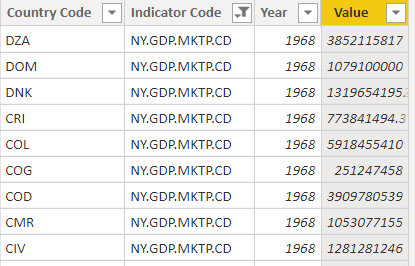Source: https://data.worldbank.org/

### Creating a measure formula using DAX

To create a measure formula using DAX, these are the steps to be followed:

Step 1: Open your Power BI Desktop app. In this Power BI Desktop, you can create a new measure in two ways.

One, you can right-click on the table’s name in which you wish to add the ‘measure’ field and select New measure.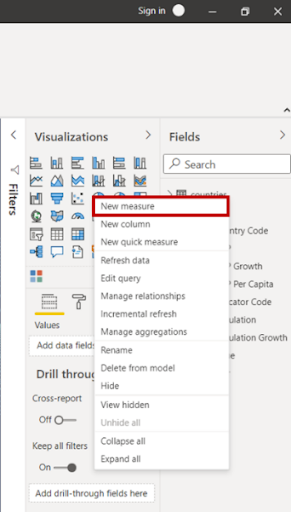Figure 1. Add a New measure by right clicking on table’s name

The second way is to the Modeling tab and click on the ‘New measure’ option.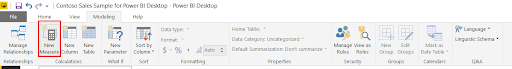Figure 2. Add a New measure using the Modeling tab

Step 2: Now, in the formula bar, begin with entering the name of your new measure to be created.

Step 3: Define the formula and its syntax elements as described below.

### DAX formula – Syntax

To understand the functioning of DAX, first, we will look into the formula and its syntax elements with the help of the example given below.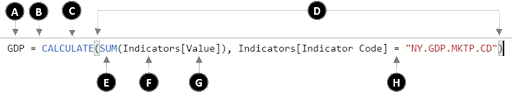Figure 3. DAX formula

where,

• Name of the new measure being created (GDP).
• The equals sign (=) is an operator which marks the start of the DAX formula and equates the two sides.
• Evaluate an expression in a modified filter context.
• The parenthesis () is used to define arguments in an expression and to define the order of evaluation. Every function must have at least one argument.
• The DAX function used to add the values of a given field (Indicator[value] and the Indicator [Indicator code]) from a table (Indicators). The function used here is known as ‘SUM.’
• It is the name of the table from which a field or column is taken in the formula (Indicators).
• It is the name of the field in the table [Indicators] from which the values are taken.For instance, the function SUM will apply itself to the values of the column or field [Value] and [Indicator Code] of the table Indicators.
• It is another operator used to equate to a specific condition. Although the syntax elements from A to G constitute the basic syntax of DAX.

Thus, in simpler words, this DAX formula commands the system to calculate the product of the sum of the values of the GDP values listed in the Indicators table where the [Indicator Code] = “NY.GDP.MKTP.CD” (i.e., GDP) and store that value in a new field or column known as GDP.

Step 4: Save the business analytics formula with its syntax elements. The newly created measure will appear in the ‘Fields’ column under the table ‘Indicators’.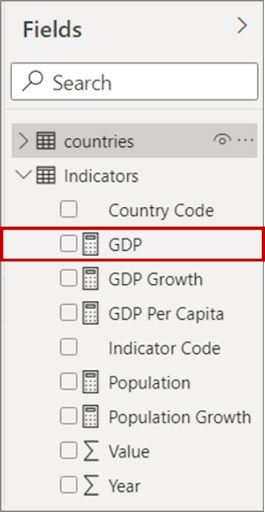Figure 4. Save the created measure in Fields under Table name

Output: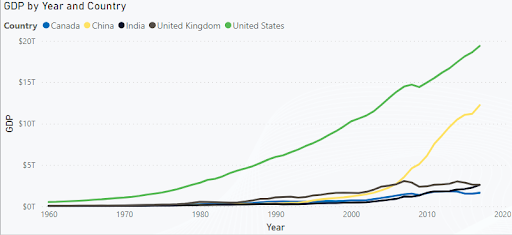Figure 5. GDP by Year and Country

Using our DAX calculated measure, we were able to plot the line diagram for different GDP business analytics values of respective countries on a year-by-year basis.

### Conclusion

DAX provides us with the flexibility and the dynamism to incorporate the worked-out formula for retrieving new information from the data already available to us in our model. DAX helps us in unlocking the full potential of the data for business analytics. DAX can also be used for the time-based comparison and the Power Pivot functionality as well.